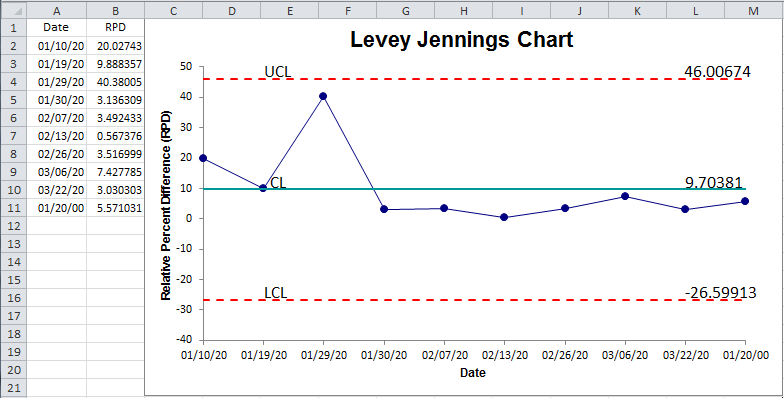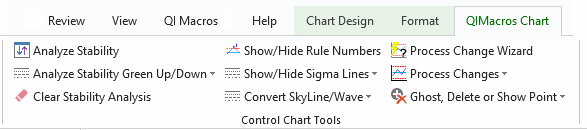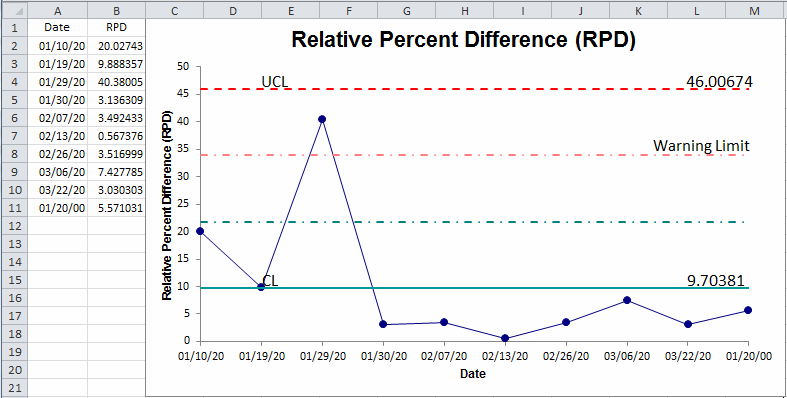# Need to Create a Precision Control Chart but Don't Know How?

## QI Macros can Create a Precision Control Chart for You. Right in Excel.

### Healthcare labs use the Precision Charts to monitor paired measures.

#### QI Macros SPC Software for Excel provides two ways to create a precision chart:

1. as a Levey Jennings Chart of Relative Percent Difference (RPD)
2. or the R Chart of an XbarR Chart of the two different measures.

### To create a Precision chart using a Levey Jennings Chart

1. First calculate the Relative Percent Difference between two sets of data. See the formula in the formula bar below and the RPD calculation results in Column D.2. Next, create a Levey Jennings chart by selecting the dates in A1:A11 and then, using the Ctrl key, select the RPD data in D1:D11. Now click on QI Macros menu > Control Charts (SPC) > Special (CUSUM, EWMA) and then Levey Jennings Stdev.
3.4. Convert the Levey Jennings chart to a Precision Chart by:
• Adjusting the Y axis to cross at zero
• Using QI Macros chart tools menu to Show the 1-2 Sigma lines5. Now you have a Precision chart. The 2-sigma line is known as the Warning Limit. In this example, one point is above the warning limit.### To create a Precision chart of the ranges between two measures:

1. Select the dates in column A and measurements in columns B and C (A1:C11)
2. Next, click on QI Macros menu, Control Charts > Variable Charts > and then the XbarR chart.3. Use QI Macros chart tools menu to Show the 1-2 Sigma lines.
4. Keep the Range Chart and discard the X Chart: